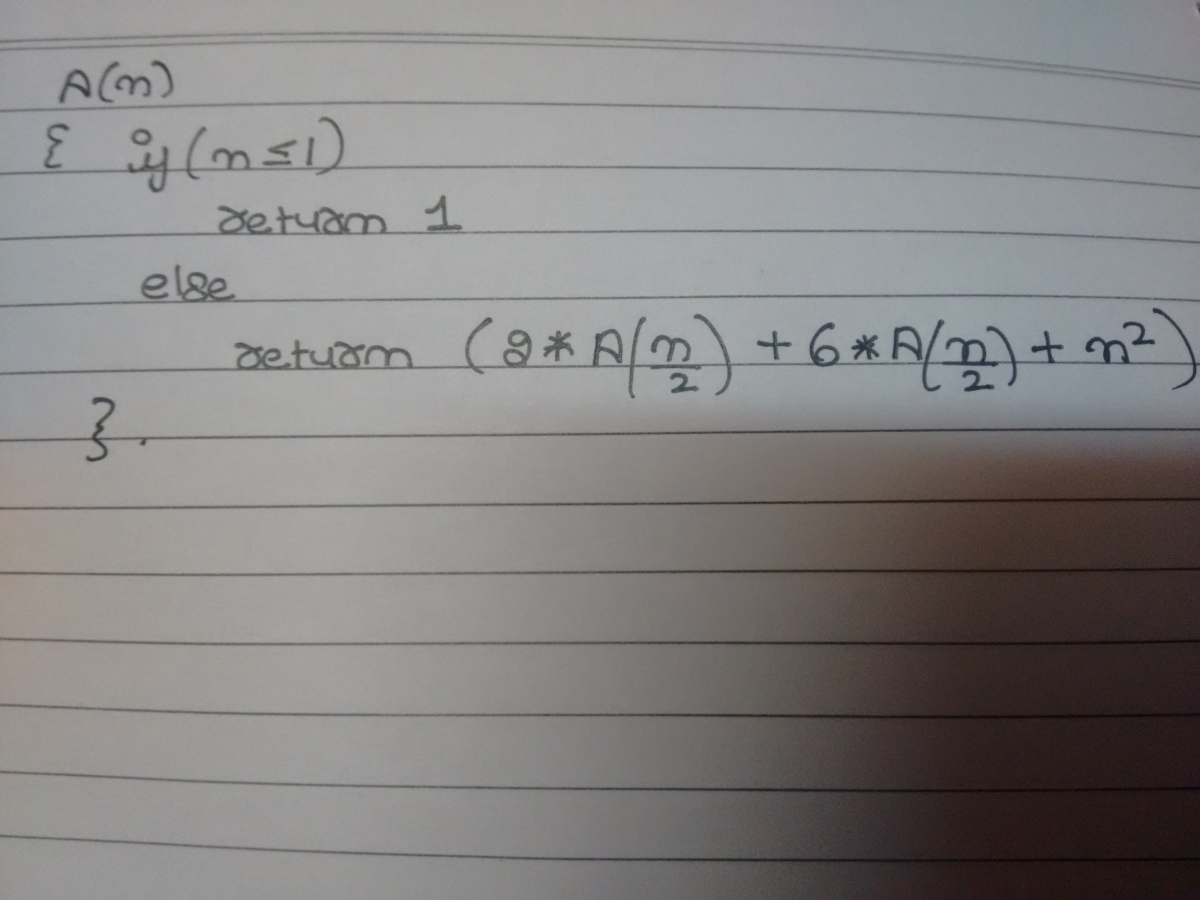##### Find the time complexity of this code snippet.Parth Sharma
18 Jul 2017 11:25 am

T (n)=T (n/2) + T (n/2) + c

C time for that addition and 2  multipliactions and 2 recursive calls are there of half size

I hope we can solve that easily

shivani
21 Jul 2017 04:14 pm

the recursive equation can be written as A(n)=8A(n/2)+ n2

Apply master theorem , then case -> a>bk

then ans is O(n3)